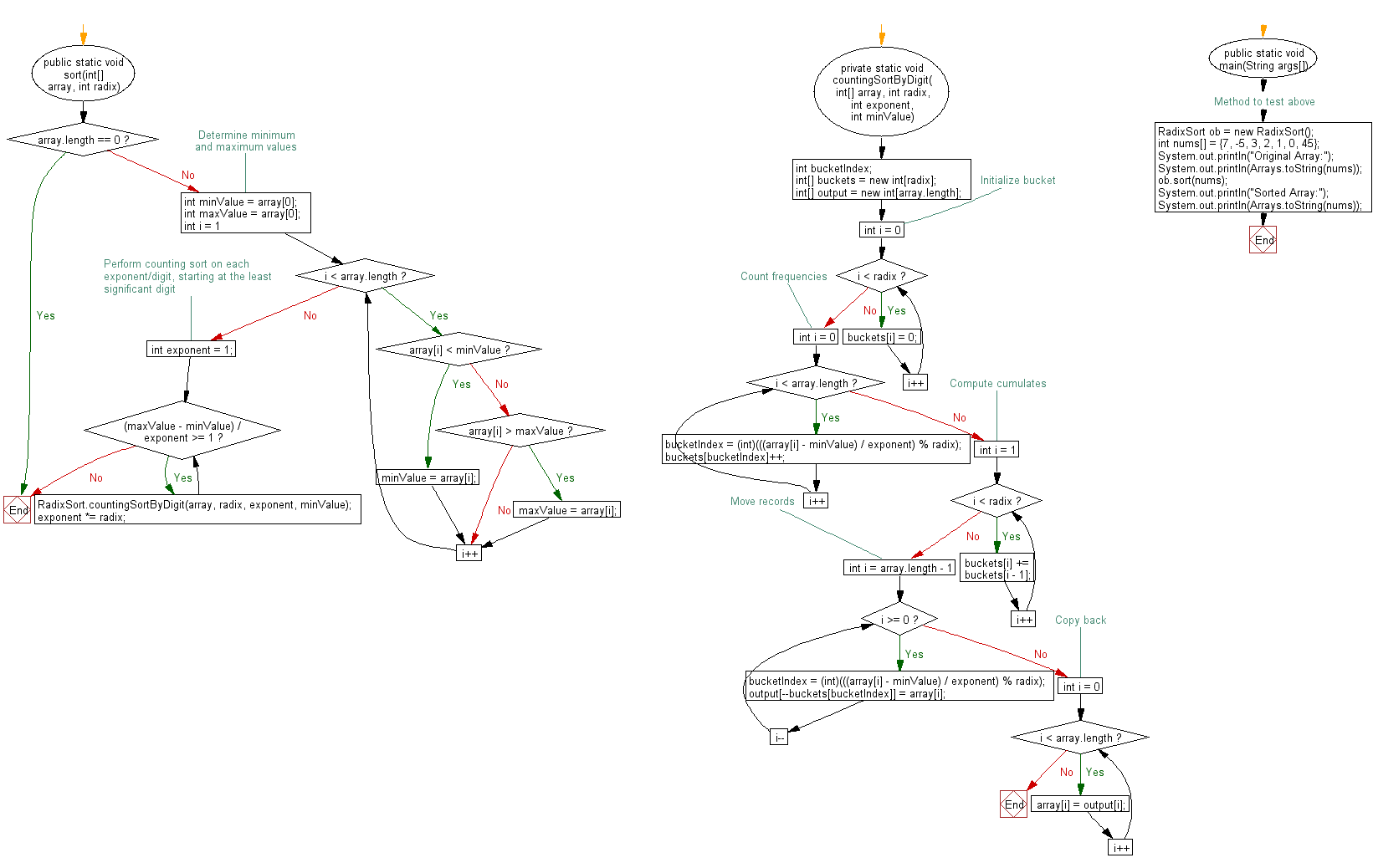﻿ Java exercises: Radix sort Algorithm - w3resource# Java Exercises: Sort an array of given integers using Radix sort Algorithm

## Java Sorting Algorithm: Exercise-3 with Solution

Write a Java program to sort an array of given integers using Radix sort Algorithm.

According to Wikipedia "In computer science, radix sort is a non-comparative integer sorting algorithm that sorts data with integer keys by grouping keys by the individual digits which share the same significant position and value".

Sample Solution:

Java Code:

``````//https://bit.ly/2MJHo7J
import java.util.Arrays;
public static void sort(int[] array) {
}

public static void sort(int[] array, int radix) {
if (array.length == 0) {
return;
}

// Determine minimum and maximum values
int minValue = array;
int maxValue = array;
for (int i = 1; i < array.length; i++) {
if (array[i] < minValue) {
minValue = array[i];
} else if (array[i] > maxValue) {
maxValue = array[i];
}
}

// Perform counting sort on each exponent/digit, starting at the least
// significant digit
int exponent = 1;
while ((maxValue - minValue) / exponent >= 1) {
}
}

private static void countingSortByDigit(
int[] array, int radix, int exponent, int minValue) {
int bucketIndex;
int[] output = new int[array.length];

// Initialize bucket
for (int i = 0; i < radix; i++) {
buckets[i] = 0;
}

// Count frequencies
for (int i = 0; i < array.length; i++) {
bucketIndex = (int)(((array[i] - minValue) / exponent) % radix);
buckets[bucketIndex]++;
}

// Compute cumulates
for (int i = 1; i < radix; i++) {
buckets[i] += buckets[i - 1];
}

// Move records
for (int i = array.length - 1; i >= 0; i--) {
bucketIndex = (int)(((array[i] - minValue) / exponent) % radix);
output[--buckets[bucketIndex]] = array[i];
}

// Copy back
for (int i = 0; i < array.length; i++) {
array[i] = output[i];
}
}
// Method to test above
public static void main(String args[])
{
int nums[] = {7, -5, 3, 2, 1, 0, 45};
System.out.println("Original Array:");
System.out.println(Arrays.toString(nums));
ob.sort(nums);
System.out.println("Sorted Array:");
System.out.println(Arrays.toString(nums));
}
}
```
```

Sample Output:

```Original Array:
[7, -5, 3, 2, 1, 0, 45]
Sorted Array:
[-5, 0, 1, 2, 3, 7, 45]
```

Flowchart:Java Code Editor:

What is the difficulty level of this exercise?

﻿

## Java: Tips of the Day

Array vs ArrayLists:

The main difference between these two is that an Array is of fixed size so once you have created an Array you cannot change it but the ArrayList is not of fixed size. You can create instances of ArrayLists without specifying its size. So if you create such instances of an ArrayList without specifying its size Java will create an instance of an ArrayList of default size.

Once an ArrayList is full it re-sizes itself. In fact, an ArrayList is internally supported by an array. So when an ArrayList is resized it will slow down its performance a bit as the contents of the old Array must be copied to a new Array.

At the same time, it's compulsory to specify the size of an Array directly or indirectly while creating it. And also Arrays can store both primitives and objects while ArrayLists only can store objects.

Ref: https://bit.ly/3o8L2KH